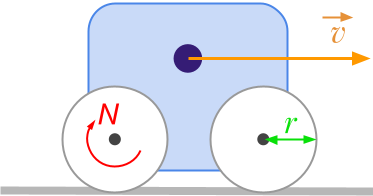👋 Hello! I'm Alphonsio the robot. Ask me a question, I'll try to answer.

# Could you explain the process to convert rpm to km/h?

To explain the process to convert an angular velocity expressed in rpm into a linear velocity expressed in km/h, let's consider the following vehicle:• The radius of the wheels is ﻿﻿ , expressed in meters [m].
• The angular velocity of the wheels is ﻿﻿ expressed in revolution per minute [rpm].
• The linear velocity of the vehicle is ﻿﻿, expressed in kilometres per hour [km/h].

Angular velocity N represents the number of turn during one minute. To get the number of revolution in one second, we just have to divide ﻿﻿ by 60. Since, one revolution is equal to ﻿﻿ radians, we can deduce the angular velocity expressed in rad/s:

﻿﻿

The formula to convert linear velocity to angular velocity is given by ﻿﻿, in our case, the result is expressed in m/s:

﻿﻿

By merging step 1 and 2, we get:

﻿﻿

To convert m/s to kilometres per hour, we need two arithmetic operations :

• convert seconds to hours (multiply m/s by 3600)
• convert meters to kilometers (divide by 1000)

The formula to convert meters per second to kilometres per hour is given by :

﻿﻿

By merging step 1, 2 and 3, we finally get :

﻿﻿

The last equation can be simplified:

﻿﻿

﻿﻿

We get the final formula from rpm to km/h:

﻿﻿

Since the diameter of the wheels is twice the radius of the wheels, the last formula can also be expressed as :

﻿﻿

More